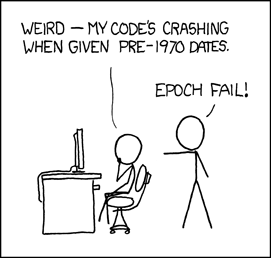# Manual Pages  — EXP

### NAME

exp, expf, expl, exp2, exp2f, exp2l, expm1, expm1f, expm1l, pow, powf, powl – exponential and power functions

### LIBRARY

Math Library (libm, -lm)

### SYNOPSIS

`#include <math.h>`

double
exp(double x);

float
expf(float x);

long double
expl(long double x);

double
exp2(double x);

float
exp2f(float x);

long double
exp2l(long double x);

double
expm1(double x);

float
expm1f(float x);

long double
expm1l(long double x);

double
pow(double x, double y);

float
powf(float x, float y);

long double
powl(long double x, long double y);

### DESCRIPTION

The exp(), expf(), and expl() functions compute the base
.Ms e exponential value of the given argument x.

The exp2(), exp2f(), and exp2l() functions compute the base 2 exponential of the given argument x.

The expm1(), expm1f(), and the expm1l() functions compute the value exp(x)-1 accurately even for tiny argument x.

The pow(), powf(), and the powl() functions compute the value of x to the exponent y.

### ERROR (due to Roundoff etc.)

The values of exp(), expm1(), exp2(integer), and pow(integer, integer) are exact provided that they are representable. Otherwise the error in these functions is generally below one ulp.

### RETURN VALUES

These functions will return the appropriate computation unless an error occurs or an argument is out of range. The functions pow(x, y), powf(x, y), and powl(x, y) raise an invalid exception and return an NaN if x < 0 and y is not an integer.

### NOTES

The function pow(x) returns x**0 = 1 for all x including x = 0, ∞, and NaN . Previous implementations of pow may have defined x**0 to be undefined in some or all of these cases. Here are reasons for returning x**0 = 1 always:
1. Any program that already tests whether x is zero (or infinite or NaN) before computing x**0 cannot care whether 0**0 = 1 or not. Any program that depends upon 0**0 to be invalid is dubious anyway since that expression's meaning and, if invalid, its consequences vary from one computer system to another.
2. Some Algebra texts (e.g.amp; Sigler's) define x**0 = 1 for all x, including x = 0. This is compatible with the convention that accepts a as the value of polynomial
```p(x) = a*x**0 + a*x**1 + a*x**2 +...+ a[n]*x**n
```

at x = 0 rather than reject a*0**0 as invalid.

3. Analysts will accept 0**0 = 1 despite that x**y can approach anything or nothing as x and y approach 0 independently. The reason for setting 0**0 = 1 anyway is this: If x(z) and y(z) are any functions analytic (expandable in power series) in z around z = 0, and if there x(0) = y(0) = 0, then x(z)**y(z) -&gt; 1 as z -&gt; 0.
4. If 0**0 = 1, then ∞**0 = 1/0**0 = 1 too; and then NaN**0 = 1 too because x**0 = 1 for all finite and infinite x, i.e., independently of x.

### STANDARDS

These functions conform to ISO/IEC 9899:1999 ("ISO C99").

### HISTORY

The exp() function appeared in AT&T v1 .

 EXP (3) April 1, 2020